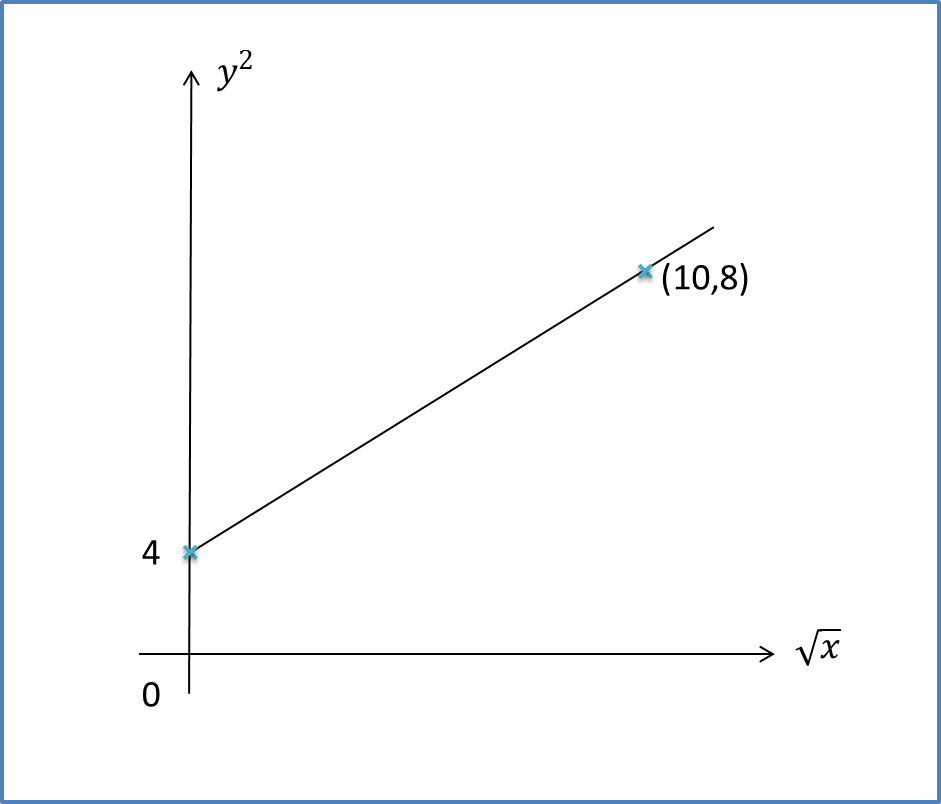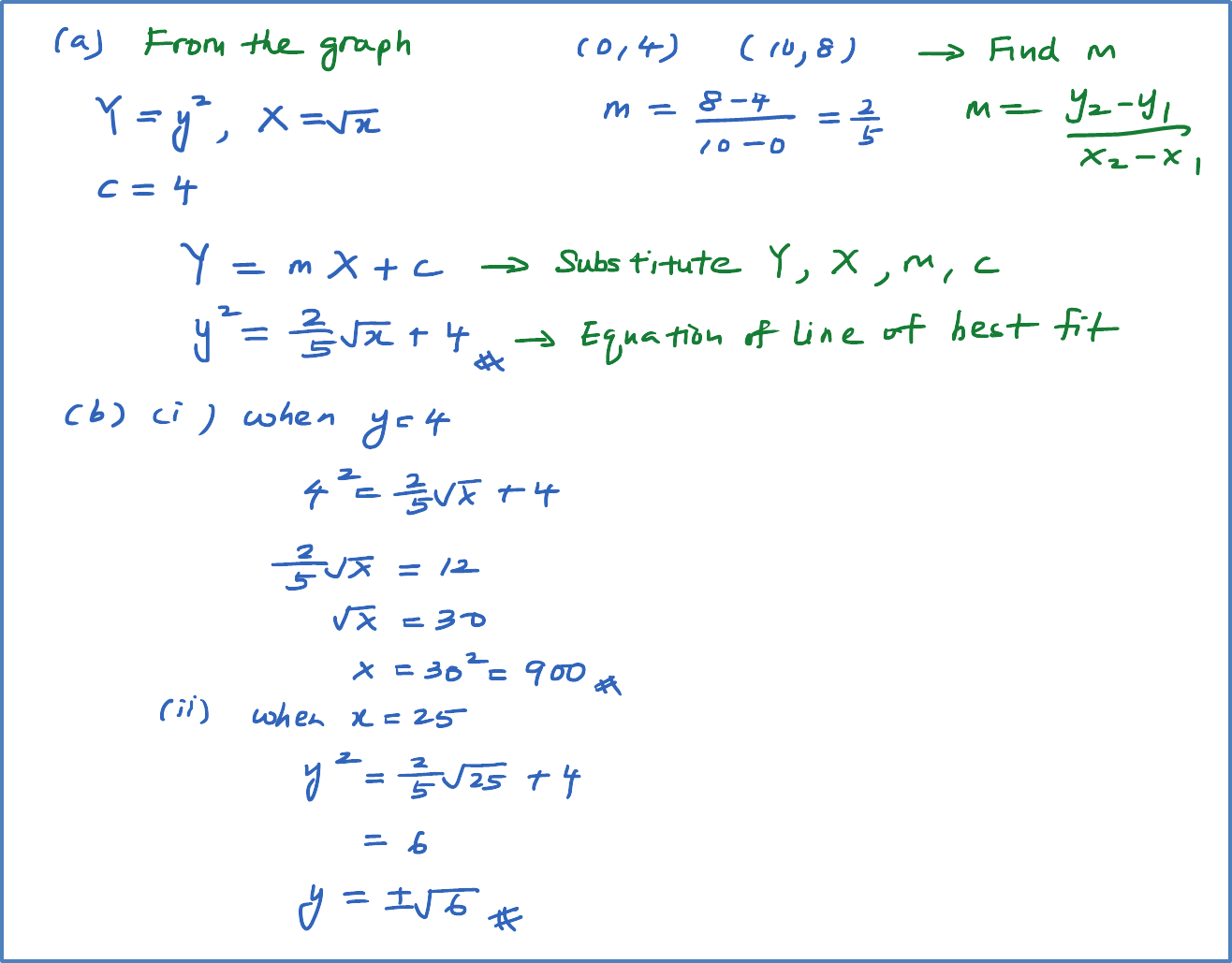\

# SPM Practice 2 (Question 1 – 3)

Question 1:
Reduce non-linear relation, $y=p{x}^{n-1}$, where k and n are constants, to linear equation.  State the gradient and vertical intercept for the linear equation obtained.
[Note : Reduce No-linear function to linear function]

Solution:Question 2:
The diagram shows a line of best fit by plotting a graph of  ${y}^{2}$against $\sqrt{x}$.1. Find the equation of the line of best fit.
2. Determine the value of
1. x when y = 4,
2. y when x = 25.
Solution:Question 3:
The diagram shows part of the straight line graph obtained by plotting$\sqrt{y}$ against ${x}^{2}$.Express y in terms of x.

Solution: